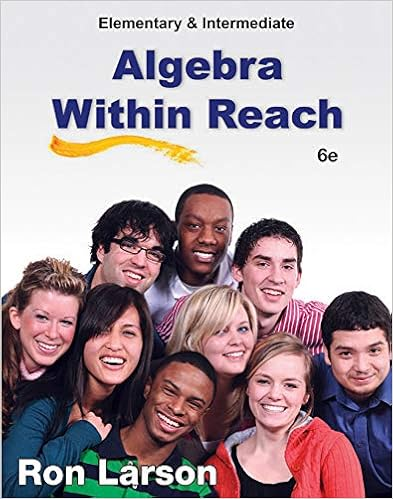# In a regression and correlation analysis if the

• Test Prep
• 76
• 80% (10) 8 out of 10 people found this document helpful

This preview shows page 65 - 69 out of 76 pages.

##### We have textbook solutions for you!
The document you are viewing contains questions related to this textbook.The document you are viewing contains questions related to this textbook.
Chapter 3 / Exercise 19
Elementary and Intermediate Algebra: Algebra Within Reach
LarsonExpert Verified
11.In a regression and correlation analysis if the coefficient of determination R2= 1, thena.SSE must also be equal to oneb.SSE must be equal to zeroc.SSE can be any positive valued.SSE must be negativeANS: B12.In a regression analysis, the regression equation is given by yˆ= 12 - 6x. If SSE = 510 and SST = 1000, then the coefficient of correlation isa.-0.7b.+0.7c.0.49d.-0.49ANS: A65
##### We have textbook solutions for you!
The document you are viewing contains questions related to this textbook.The document you are viewing contains questions related to this textbook.
Chapter 3 / Exercise 19
Elementary and Intermediate Algebra: Algebra Within Reach
LarsonExpert Verified
13.One of the measures of the accuracy of the regression model is called the coefficient ofa.regressionb.determinationc.statistical variabilityd.statistical importanceANS: B14.Regression analysis was applied between demand for a product (y) and the price of the product (x), and the following estimated regression equation was obtained: xy10120ˆ. Based on the above estimated regression equation, if price is increased by 2 units, then demand is expected toa.increase by 120 unitsb.increase by 100 unitsc.increase by 20 unitsd.decrease by 20 unitsANS: D15.If the correlation coefficient is positive, then the slope of the regression linea.must also be positiveb.can be either negative or positivec.can be zerod.cannot be zeroANS: AExhibit 12-1The following information regarding a dependent variable (y) and an independent variable (x) is provided.yx4231446385SSE = 6 and SST = 16.16.Refer to Exhibit 14-1. The least squares estimate of the slope isa.1b.2c.3d.4ANS: A17. Refer to Exhibit 12-1. The least squares estimate of the yintercept isa.1b.2c.3d.4ANS: B66
18.Refer to Exhibit 12-1. The sample coefficient of determination isa.0.7096b.- 0.7906c.0.625d.0.375ANS: C19.Refer to Exhibit 12-1. The sample coefficient of correlation isa.0.7906b.- 0.7906c.0.625d.0.375ANS: A20.Refer to Exhibit 12-1. The mean squared error (MSE) isa.1b.2c.3d.4ANS: B21. Refer to Exhibit 12-1. The standard error of the estimate, s, is a.1b.1.4142c.1.7321d.2ANS: BExhibit 12-2Regression analysis was applied between sales data (yin \$1,000s) and advertising expenditures (xin \$100s). A random sample of 17 observations led to the following information:ANOVAdfSSMSFRegression225ErrorTotal300CoefficientsStandard Errort StatIntercept12Expenditure1.80.268322.Refer to Exhibit 12-2. If \$3,000 is spent on advertising, what are the predicted sales?a.\$66,000b.\$5,412c.\$66067
d.\$660,000ANS: A23.Refer to Exhibit 12-2. If \$100 additional dollars is spent on advertising, then the predicted sales willa.increase by \$180b.increase by \$1,800c.increase by \$18,000d.Will remain unchangedANS: B
•••